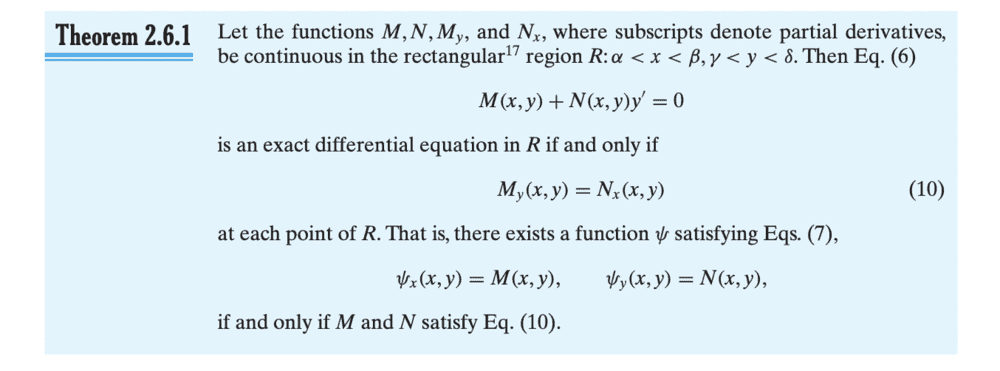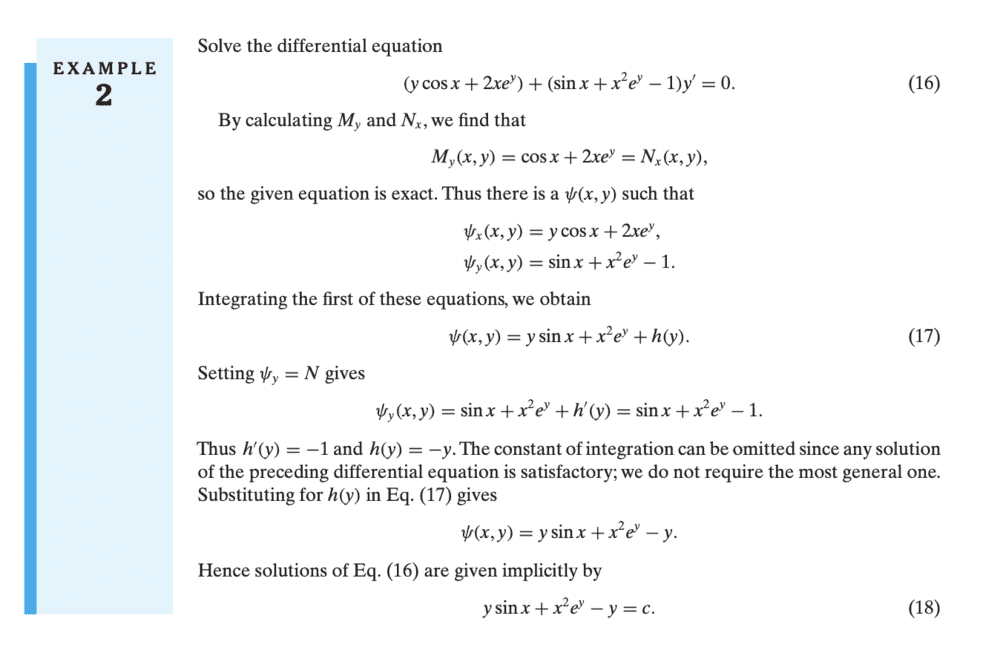# How do I solve this differential equation?

• potatocake
In summary,You are looking for a function f(x,y) such that \begin{align*}\frac{\partial f}{\partial x} &= x \cos(y) + x^2 +y \\\frac{\partial f}{\partial y} &= x + y^2 -\frac12 x^2 \sin y\end{align*} The solution is then given implicitly by f(x,y(x))) = C.f

#### potatocake

Homework Statement
(x cos(y) + x^2 +y ) dx + (x + y^2 - (x^2)/2 sin y ) dy = 0
Relevant Equations
(x cos(y) + x^2 +y ) dx = - (x + y^2 - (x^2)/2 sin y ) dy
(x cos(y) + x2 +y ) dx = - (x + y2 - (x2)/2 sin y ) dy
I integrated both sides
1/2x2cos(y) + 1/3 x3+xy = -xy - 1/3y3+x2cos(y)

Then
I get x3 + 6xy + y3 = 0

Am I doing the calculations correctly?
Do I need to solve it in another way?

•Delta2
You are looking for a function $f(x,y)$ such that \begin{align*} \frac{\partial f}{\partial x} &= x \cos(y) + x^2 +y \\ \frac{\partial f}{\partial y} &= x + y^2 -\frac12 x^2 \sin y \end{align*} The solution is then given implicitly by $f(x,y(x))) = C$.

Are you familiar with how to solve such a system?

•Delta2 and WWGD
You are looking for a function $f(x,y)$ such that \begin{align*} \frac{\partial f}{\partial x} &= x \cos(y) + x^2 +y \\ \frac{\partial f}{\partial y} &= x + y^2 -\frac12 x^2 \sin y \end{align*} The solution is then given implicitly by $f(x,y(x))) = C$.

Are you familiar with how to solve such a system?
Then do I have to solve two different differential equations?

the first thing i notice is that if i take the partial of the first equation with respect to y it is equal to the partial of the second equation with respect to x. I think this means the system is "exact". My differential equations are a bit rusty. To solve this system, you may need to review exact equations in yout textbook,

the first thing i notice is that if i take the partial of the first equation with respect to y it is equal to the partial of the second equation with respect to x. I think this means the system is "exact". My differential equations are a bit rusty. To solve this system, you may need to review exact equations in yout textbook,
Correct. It implies there is f(x,y) with f_x , f_y equal to the respective equations.

This is a class of ODE known as exact equations in this text

Here is the theorem that gives the solution and a corresponding example from the textonce you get the hang of the process it becomes pretty simple actually.

This is a class of ODE known as exact equations in this text

Here is the theorem that gives the solution and a corresponding example from the text

View attachment 281782

View attachment 281783
once you get the hang of the process it becomes pretty simple actually.
I always wondered if it is a coincidence that these are precisely the Cauchy-Riemann equations.